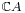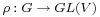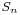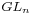- F_un mathematics - http://cage.ugent.be/~kthas/Fun -

F_un and group representations

Posted By javier On October 12, 2008 @ 3:19 pm In featured,research | 4 Comments

As a disclaimer, what follows are just some personal ramblings I came up with. It is possible that something, or even all of what follows is either well known or just plainly stupid. I claim no originality for anything below, so use it at your own risk…

In Connes-Consani paper On the notion of geometry over, the category of finite abelian groups receives a prominent use in order to modify SoulÃ©’s notion of gadget over. In his exposition for undergraduates , Lieven conceals the functor of points and the algebra bit of the gadget in terms of the so-called maxi-functor, mini-functor and nano-functor (good thing he doesn’t mention any shuffles or put too much stress on the classic situation, or he might have been tempted of calling them iFunctors and we’d be being sued by Apple right now). These functors are associated to the contruction of the corresponding group rings  with base rings either the integers or the complex numbers. He also mentions as something trivial the decomposition of the groupringas a direct sum of copies of the field, something that follows from the fact that the group algebra of a finite group is always semisimple (which is the statement of the Maschke’s Theorem ) and a straightforward use of Artin-Wedderburn Structure Theorem  for (artinian) semisimple rings.

Thinking about these results, and their relation with representation theory of groups , I started digressing about my own approach to the field. While it seems that in Antwerp they teach some representation theory of groups during the bachelor years, first thing I ever did having to do with finding concrete realization of groups was more related to Cayley’s theorem , and permutation groups, which is perhaps reasonable taking into account that the course I took was aiming to explain some basics of Galois Theory .

Making the long story short, we may think of two basic ways of representing (finite) groups. One of them, with a more geometrical flavor, is looking for a (finite dimensional) vector spaceand trying to describe our groupinside the groupof automorphisms ofby looking for group homomorphisms, also called linear representations. The other, set-theoretical one, consists on looking for (finite) setsendowed with a group action  of, also called-sets, that hopefully allow us to describeas a group of permutations.

There are many reasons to think that both approaches should have similar properties, after all we are always trying to describe the same object, however, we can give a different meaning to this relation under the light onmaths. When we look for linear representations of our group, we are automatically making a choice, not only on the vector space, but on the field over which we consider our vector space. We can look for complex linear representations, for real linear representations, for rational linear representations and so on. In particular, we might take representations defined over finite fields, diving into what is called modular representation theory . This theory behaves exactly as in the characteristic 0 case for most fields (in virtue again of Maschke’s theorem, whenever the characteristic of the field is not a divisor of the order of our group we’ll be back into the completely reducible situation), with some weird phenomena showing up in the exceptional cases. But now, since we have a new idea of a field, we might try to study linear representations over our dearest. What do we get then? Well, since the F_un folklore  tells us that vector spaces overare just sets, with cardinality corresponding to the dimension, and the Tits argument on Chevalley groups  points at the permutation group, which is the Weyl group  of, as the corresponding general linear group, the-mantras will tell us that linear representations of a group over the field with one elements are precisely the permutation representations!

So, the old set-theoretical approach to representation theory of groups can be seen just as a degenerate case of the general theory of linear representations, adding a new line to our folkloric dictionary, and giving some common ground that explain the similarities between these two theories.

PS: I remember reading a while ago some dissertation in which the linear and the set theoretical approaches to representation theory where discussed and sort of tied-up together. Unfortunately I cannot remember who was the author of it. Only thing I remember is that it was written in the form of a dialog between two mathematicians, each of them defending his own way of constructing representations of the group. Any reference to this text or any clues about his author will be very welcome!

Update: The text I was thinking about is contained in John Baez’s This Week’s Finds in Mathematical Physics 252  (28th May 2007). The mathematicians involved in the discussion are Georg Frobenius  standing for the linear representations, and William Burnside  (as the G-sets champ. Certainly worth re-reading it!

This series continues here  where we try to make sense of noncommutative geometry over.

URL to article: http://cage.ugent.be/~kthas/Fun/index.php/f_un-and-group-representations.html

URLs in this post:

 Image: http://cage.ugent.be/~kthas/Fun/index.php/connes-consani2008.html

 group rings: http://en.wikipedia.org/wiki/Group_ring

 Maschke’s Theorem: http://en.wikipedia.org/wiki/Maschke%27s_theorem

 Artin-Wedderburn Structure Theorem: http://en.wikipedia.org/wiki/Artin-Wedderburn_theorem

 representation theory of groups: http://en.wikipedia.org/wiki/Group_representation

 Cayley’s theorem: http://en.wikipedia.org/wiki/Cayley_group

 Galois Theory: http://en.wikipedia.org/wiki/Galois_theory

 group action: http://en.wikipedia.org/wiki/Group_action

 modular representation theory: http://en.wikipedia.org/wiki/Modular_representation_theory

 the F_un folklore: http://cage.ugent.be/~kthas/Fun/index.php/the-f_un-folklore.html

 the Tits argument on : http://cage.ugent.be/~kthas/Fun/index.php/mathematical-phantoms.html

 Weyl group: http://en.wikipedia.org/wiki/Weyl_group

 This Week’s Finds in Mathematical Physics 252: http://math.ucr.edu/home/baez/week252.html

 Georg Frobenius: http://en.wikipedia.org/wiki/Ferdinand_Georg_Frobenius

 William Burnside: http://en.wikipedia.org/wiki/William_Burnside

 here: http://cage.ugent.be/~kthas/Fun/index.php/towards-noncommutative-f_un-geometry.html